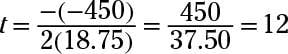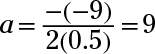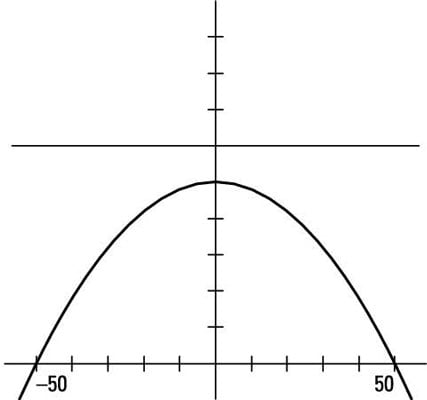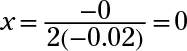##### Algebra II All-in-One For DummiesQuadratic equations lend themselves to modeling situations that happen in real life, such as the rise and fall of profits from selling goods, the decrease and increase in the amount of time it takes to run a mile based on your age, and so on.

The wonderful part of having something that can be modeled by a quadratic is that you can easily solve the equation when set equal to zero and predict the patterns in the function values.

The vertex and x-intercepts are especially useful. These intercepts tell you where numbers change from positive to negative or negative to positive, so you know, for instance, where the ground is located in a physics problem or when you’d start making a profit or losing money in a business venture.

The vertex tells you where you can find the absolute maximum or minimum cost, profit, speed, height, time, or whatever you’re modeling.

## Sample question

1. In 1972, you could buy a Mercury Comet for about \$3,200. Cars can depreciate in value pretty quickly, but a 1972 Comet in pristine condition may be worth a lot of money to a collector today.

Let the value of one of these Comets be modeled by the quadratic function v(t) = 18.75t2 – 450t + 3,200, where t is the number of years since 1972. When is the value of the function equal to 0 (what is an x-intercept), what was the car’s lowest value, and what was its value in 2010?

The car’s value never dropped to 0, the lowest value was \$500, and the car was worth \$13,175 in the year 2010. In this model, the y-intercept represents the initial value. When t = 0, the function is v(0) = 3,200, which corresponds to the purchase price.

Find the x-intercepts by solving 18.75t2 –450t + 3,200 = 0. Using the quadratic formula (you could try factoring, but it’s a bit of a challenge and, as it turns out, the equation doesn’t factor), you get –37,500 under the radical in the formula. You can’t get a real-number solution, so the graph has no x-intercept. The value of the Comet doesn’t ever get down to 0.

Find the lowest value by determining the vertex. Using the formula,This coordinate tells you that 12 years from the beginning (1984 — add 12 to 1972), the value of the Comet is at its lowest. Replace the t’s in the formula with 12, and you get v(12) = 18.75(12)2 – 450(12) + 3,200 = 500.

The Comet was worth \$500 in 1984. To find the value of the car in 2010, you let t = 38, because the year 2010 is 38 years after 1972. The value of the car in 2010 is v(38) = 18.75(38)2 – 450(38) + 3,200 = \$13,175.

## Practice questions

1. The height of a ball t seconds after it’s thrown into the air from the top of a building can be modeled by h(t) = –16t2 + 48t + 64, where h(t) is height in feet. How high is the building, how high does the ball rise before starting to drop downward, and after how many seconds does the ball hit the ground?

2. The profit function telling Georgio how much money he will net for producing and selling x specialty umbrellas is given by P(x) = –0.00405x2 + 8.15x – 100.

What is Georgio’s loss if he doesn’t sell any of the umbrellas he produces, how many umbrellas does he have to sell to break even, and how many does he have to sell to earn the greatest possible profit?

3. Chip ran through a maze in less than a minute the first time he tried. His times got better for a while with each new try, but then his times got worse (he took longer) due to fatigue.

The amount of time Chip took to run through the maze on the ath try can be modeled by T(a) = 0.5a2 – 9a + 48.5. How long did Chip take to run the maze the first time, and what was his best time?

4. A highway underpass is parabolic in shape. If the curve of the underpass can be modeled by h(x) = 50 – 0.02x2, where x and h(x) are in feet, then how high is the highest point of the underpass, and how wide is it?

Following are answers to the practice questions:

1. The building is 64 feet tall, the ball peaks at 100 feet, and it takes 4 seconds to hit the ground.

The ball is thrown from the top of the building, so you want the height of the ball when t = 0. This number is the initial t value (the y-intercept). When t = 0, h = 64, so the building is 64 feet high.

The ball is at its highest at the vertex of the parabola. Calculating the t value, you get that the vertex occurs where t = 1.5 seconds. Substituting t = 1.5 into the formula, you get that h = 100 feet.

The ball hits the ground when h = 0. Solving –16t2 + 48t + 64 = 0, you factor to get –16(t – 4)(t + 1) = 0. The solution t = 4 tells you when the ball hits the ground.

The t = –1 represents going backward in time, or in this case, where the ball would have started if it had been launched from the ground — not the top of a building.

2. Georgio loses \$100 (earns –\$100) if he sells 0, needs to sell 13 to break even, and can maximize profits if he sells 1,006 umbrellas.

If Georgio sells no umbrellas, then x = 0, and he makes a negative profit (loss) of \$100. The break-even point comes when the profit changes from negative to positive, at an x-intercept. Using the quadratic formula, you get two intercepts: at x = 2,000 and x is approximately 12.35.

The first (smaller) x-intercept is where the function changes from negative to positive. The second is where the profit becomes a loss again (too many umbrellas, too much overtime?). So, 13 umbrellas would yield a positive profit — he’d break even (have zero profit).

The maximum profit occurs at the vertex. Using the formula for the x-value of the vertex, you get that x is approximately 1,006.17. Substituting 1,006 into the formula, you get 4,000.1542; then substituting 1,007 into the formula, you get 4,000.15155.

You see that Georgio gets slightly more profit with 1,006 umbrellas, but that fraction of a cent doesn’t mean much. He’d still make about \$4,000.

3. Chip took 40 seconds the first time; his best time was 8 seconds.

Because the variable a represents the number of the attempt, find T(1) for the time of the first attempt. T(1) = 40 seconds. The best (minimum) time is at the vertex. Solving for the a value (which is the number of the attempt),He had the best time on the ninth attempt, and T(9) = 8.

4. The underpass is 50 feet high and 100 feet wide.The highest point occurs at the vertex:The x-coordinate of the vertex is 0, so the vertex is also the y-intercept, at (0, 50). The two x-intercepts represent the endpoints of the width of the overpass. Setting 50 – 0.02x2 equal to 0, you solve for x and get x = 50, –50. These two points are 100 units apart — the width of the underpass.